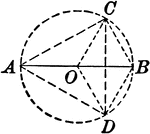### Circle With Inscribed Triangle and Radii

Illustration showing a circle with an inscribed triangle and radii.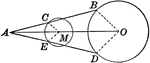### Midpoint of Straight Line Drawn From an Exterior point to Circumference

Illustration showing a line with a midpoint drawn from a given exterior point to a given circumference.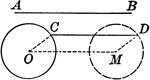### Straight Line Moving to Two circles

Illustration showing a line that remains parallel to a given line, and touches at one end a given circumference.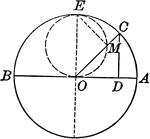### Circle Made by Ends of a Line Touching Two Fixed Lines Perpendicular to Each Other

Illustration showing a line that moves so that its ends constantly touch two fixed lines which are perpendicular…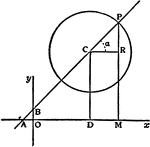### Straight Line

The straight line is the simplest type of locus and the simplest first degree equation.